# Railway

Between points A, B, whose horizontal distance is 1.5 km railway line has 8promile climb. Between points B, C with horizontal distance of 900 m is climb 14promile. Calculate differences of altitudes between points A and C.

x =  24.6 m

### Step-by-step explanation:Did you find an error or inaccuracy? Feel free to write us. Thank you!Tips to related online calculators
Do you want to convert length units?

#### You need to know the following knowledge to solve this word math problem:

We encourage you to watch this tutorial video on this math problem:

## Related math problems and questions:

• Mountain railway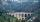Height difference between points A, B of railway line is 38.5 meters, their horizontal distance is 3.5 km. Determine average climb in permille up the track.
• Climb in percentageThe height difference between points A and B is 475 m. Calculate the percentage of route climbing if the horizontal distance places A, B is 7.4 km.
• The railway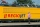The railway line has a gradient of 12 per mille. How many meters will it ascend to a horizontal distance of 4 km?
• RailwayRailway line had on 5.8 km segment climb 9 permille. How many meters track ascent?
• ClimbFor horizontal distance 4.2 km road rise by 6.3 m. Calculate the road pitch in ‰ (permille, parts per thousand).
• RailwaysRailways climb 7.4 ‰. Calculate the height difference between two points on the railway distant 3539 meters.
• Slope of trackCalculate the average slope (in permille and even in degrees) of the rail tracks between Prievidza (309 m AMSL) and Nitrianske Pravno (354 m AMSL), if the track is 11 km long.
• RiverCalculate how many permille river Dunaj average falls, if on section long 957 km flowing water from 1454 m AMSL to 101 m AMSL.
• Cablecar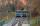Funicular on Petrin (Prague) was 408 meters long and overcomes the difference 106 meters in altitude. Calculate the angle of climb.
• AMSL and skiingTomas skis from point A (3200m above sea level to place B. Hill has 20% descent. The horizontal distance between the start and finish is 2,5km. At what altitude is point B?
• SSA and geometryThe distance between the points P and Q was 356 m measured in the terrain. The PQ line can be seen from the viewer at a viewing angle of 107° 22 '. The observer's distance from P is 271 m. Determine the viewing angle of P and observer.
• Map - climb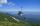On the map of High Tatras in scale 1:11000 are cable car stations in the Tatranska Lomnica and in the Skalnate Pleso with distance 354.6 mm. Altitude of this stations are 949 m and 1760 m. What is average angle of climb of this cable car track?
• A drone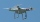A flying drone aimed the area for an architect. He took off perpendicularly from point C to point D. He was at the height of 300 m above ABC's plane. The drone from point D pointed at a BDC angle of 43°. Calculate the distance between points C and B in meBetween cities A and B is route 13 km long of stúpanie average 7‰. Calculate the height difference of cities A and B.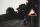A 5 km long road begins at an altitude 500 meters above sea level and ends at an altitude of 521 ASL. How many permille road rises?Find the elevation difference of the cable car when it rises by 67 per mille and the rope length is 930 m.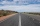The road has a gradient of 8%. How many meters will the road rise on a horizontal distance of 400m?